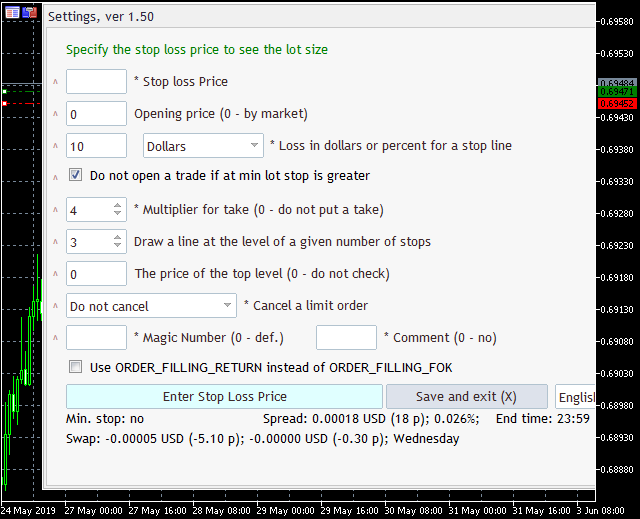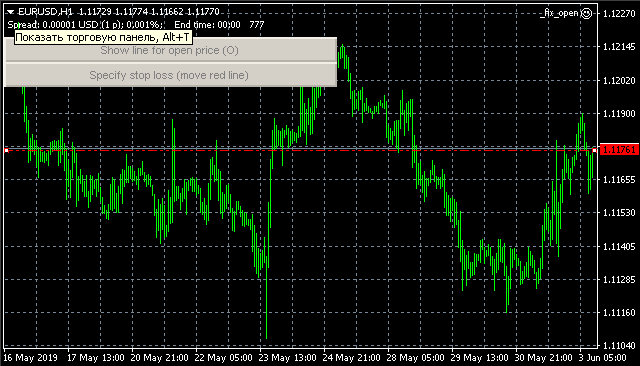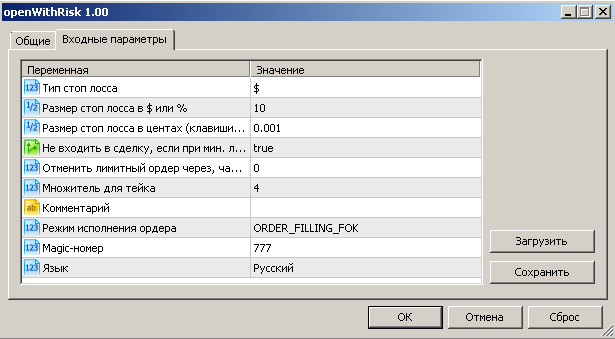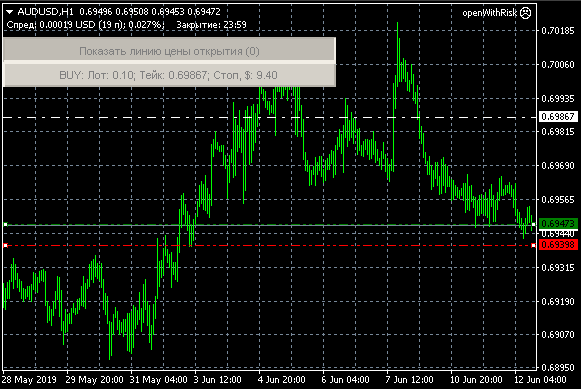# 开发一个跨平台的EA交易来根据风险设置止损和获利

### 简介

N 是由交易者选择的。为了符合这一规则，人们应该正确地计算出交易手数的值。

### 任务定义

Gerchik 和其他成功的交易者建议使用获利，这至少是你止损的3倍。也就是说，如果你用 40 个点的止损分，那么获利至少应该是 120 个点。如果价格没有太多机会达到这个水平，你最好不要进入这个交易。

EA 交易的形式. 在进行EA开发之前，我们需要决定它的运行模式。这可能是最困难的任务，• 每次启动时需要重新配置EA
• 每次启动时需要从一个设置文件上载设置
• 需要更改EA代码中的默认设置并重新编译它### 输入参数Stop Loss value in cents (keys 7 and 8)（以分表示的止损值 (按键 7 和 8)）. 另一个止损相关的参数是: "Stop Loss value in cents (keys 7 and 8)".

No deal entry if with min. lot the risk is greater than specified.（如果使用最小手数风险还是大于指定的，就不交易） 因为交易入场手数是根据 " Stop Loss value in \$ or %" 参数自动计算的, 当最低允许手数会导致每笔交易的风险高于规定值时，可能会出现这种情况。

Cancel limit order after, hours.（取消限价订单的小时数）除了进入市场外，EA还支持根据指定价格下限价订单。此参数允许限制订单寿命，

Tale Profit multiplier.（获利倍数）如果您的交易具有特定的获利与止损比率，此参数可用于根据您的规则配置自动获利设置，

• 更改EA订单的幻数
• 为订单设置注释
• 选择界面语言：英语或俄语（默认）

### 开启仓位函数

```#ifdef __MQL5__
//MQL5 代码
#else
//MQL4 代码

```// 仓位开启函数中可能的订单类型
enum TypeOfPos
{
MY_SELL,
MY_SELLSTOP,
MY_SELLLIMIT,
MY_SELLSLTP,
};

// 为 MT5 选择交易执行类型
#ifdef __MQL5__
enum TypeOfFilling //交易执行类型
{
FOK,//ORDER_FILLING_FOK
RETURN,// ORDER_FILLING_RETURN
IOC,//ORDER_FILLING_IOC
};
input TypeOfFilling  useORDER_FILLING_RETURN=FOK; //订单执行模式

/*

*/
bool pdxSendOrder(TypeOfPos mytype, double price, double sl, double tp, double volume, ulong position=0, string comment="", string sym="", datetime expiration=0){
if( !StringLen(sym) ){
sym=_Symbol;
}
int curDigits=(int) SymbolInfoInteger(sym, SYMBOL_DIGITS);
if(sl>0){
sl=NormalizeDouble(sl,curDigits);
}
if(tp>0){
tp=NormalizeDouble(tp,curDigits);
}
if(price>0){
price=NormalizeDouble(price,curDigits);
}else{
#ifdef __MQL5__
#else
MqlTick latest_price;
SymbolInfoTick(sym,latest_price);
if( mytype == MY_SELL ){
}else if( mytype == MY_BUY ){
price=latest_price.bid;
}
在画线之前#endif
}
#ifdef __MQL5__
switch(mytype){
break;
break;
break;
break;
case MY_SELL:
type=ORDER_TYPE_SELL;
break;
case MY_SELLSLTP:
type=ORDER_TYPE_SELL;
break;
case MY_SELLSTOP:
type=ORDER_TYPE_SELL_STOP;
break;
case MY_SELLLIMIT:
type=ORDER_TYPE_SELL_LIMIT;
break;
}

ZeroMemory(mrequest);

mrequest.action = action;
mrequest.sl = sl;
mrequest.tp = tp;
mrequest.symbol = sym;
if(expiration>0){
mrequest.type_time = ORDER_TIME_SPECIFIED_DAY;
mrequest.expiration = expiration;
}
if(position>0){
mrequest.position = position;
}
if(StringLen(comment)){
mrequest.comment=comment;
}
if(price>0){
mrequest.price = price;
}
if(volume>0){
mrequest.volume = volume;
}
mrequest.type = type;
mrequest.magic = EA_Magic;
switch(useORDER_FILLING_RETURN){
case FOK:
mrequest.type_filling = ORDER_FILLING_FOK;
break;
case RETURN:
mrequest.type_filling = ORDER_FILLING_RETURN;
break;
case IOC:
mrequest.type_filling = ORDER_FILLING_IOC;
break;
}
mrequest.deviation=100;
}
if(OrderSend(mrequest,mresult)){
if(mresult.retcode==10009 || mresult.retcode==10008){
switch(type){
break;
case ORDER_TYPE_SELL:
//                     Alert("Order Sell #:",mresult.order," sl",sl," tp",tp," p",price," !!");
break;
}
}else{
//               Alert("Order Modify SL #:",mresult.order," sl",sl," tp",tp," !!");
}
return true;
}else{
msgErr(GetLastError(), mresult.retcode);
}
}
#else
switch(mytype){
break;
break;
break;
case MY_SELL:
type=OP_SELL;
break;
case MY_SELLSTOP:
type=OP_SELLSTOP;
break;
case MY_SELLLIMIT:
type=OP_SELLLIMIT;
break;
}

if(OrderSend(sym, type, volume, price, 100, sl, tp, comment, EA_Magic, expiration)<0){
msgErr(GetLastError());
}else{
switch(type){
break;
case OP_SELL:
Alert("Order Sell sl",sl," tp",tp," p",price," !!");
break;
}
return true;
}

在画线之前#endif
return false;
}```

### EA 界面的编程

• 一条将包含当前美元价差、点数和价格百分比，以及交易品种交易结束时间的注释；
• 在与当前价格的价差距离处显示一条水平线，用于放置止损；
• "Show (0) open price line" 按钮将被添加，这将在订单打开价格处显示绿色水平线；
• 打开具有指定参数的订单需要另一个按钮。

```/*
在图表注释中显示点差和交易结束时间的数据
*/
string msg="";

// 取得交易品种的点差

// 如果市场没有关闭，显示点差信息
if( !isClosed ){
}else{
}
}

// 如果我们可以确定的话，显示市场收盘时间
if(StringLen(time_info)){
}

Comment(msg);
}```

getmespread 将在 EA 初始化 (OnInit) 以及每一新报价 (OnTick) 时调用。

getmespread 中, 我们使用了5个全局 EA 变量: lastme, isClosed, time_info, currencyS, curPoint.

lastme 变量保存 Ask, Bid 和 Last 报价的数据，变量的内容在 OnInitOnTick 函数中使用下面的指令更新:

`SymbolInfoTick(_Symbol,lastme);`

```  isClosed=false;
// 取得当前日期
TimeToStruct(TimeCurrent(), curDay);
// 取得今日的交易品种交易时间
if(SymbolInfoSessionTrade(_Symbol, (ENUM_DAY_OF_WEEK) curDay.day_of_week, 0, dfrom, dto)){
time_info="";
TimeToStruct(dto, curEndTime);
TimeToStruct(dfrom, curStartTime);

isEndTime=true;
string tmpmsg="";
tmp_val=curEndTime.hour;
if(tmp_val<10){
}
tmp_val=curEndTime.min;
if(tmp_val<10){
}
if(curEndTime.hour==curDay.hour){
if(tmp_val>curDay.min){
}else{
isClosed=true;
}
}else{
if(curEndTime.hour==0){
}else{
if( curEndTime.hour>1 && (curDay.hour>curEndTime.hour || curDay.hour==0)){
isClosed=true;
}else if(curDay.hour<curStartTime.hour ){
isEndTime=false;
isClosed=true;
}else if(curDay.hour==curStartTime.hour && curDay.min<curStartTime.min ){
isEndTime=false;
isClosed=true;
}
}
}

if(isEndTime){
}else{
}
}```

currencyS变量将存储用于当前金融工具利润计算的货币,货币可以:

`currencyS=SymbolInfoString(_Symbol, SYMBOL_CURRENCY_PROFIT);`

`curPoint=SymbolInfoDouble(_Symbol, SYMBOL_POINT);`

```  // 如果图表上有止损和开仓价格线
// 把价格加到全局变量中
if(ObjectFind(0, exprefix+"_stop")>=0){
draw_stop=ObjectGetDouble(0, exprefix+"_stop", OBJPROP_PRICE);
if(ObjectFind(0, exprefix+"_open")>=0){
draw_open=ObjectGetDouble(0, exprefix+"_open", OBJPROP_PRICE);
}
// 否则创建整个 EA 交易的 UI
}else{
draw_open=lastme.bid;
ObjectCreate(0, exprefix+"_stop", OBJ_HLINE, 0, 0, draw_stop);
ObjectSetInteger(0,exprefix+"_stop",OBJPROP_SELECTABLE,1);
ObjectSetInteger(0,exprefix+"_stop",OBJPROP_SELECTED,1);
ObjectSetInteger(0,exprefix+"_stop",OBJPROP_STYLE,STYLE_DASHDOTDOT);
ObjectSetInteger(0,exprefix+"_stop",OBJPROP_ANCHOR,ANCHOR_TOP);

// 其它界面元素
}```

```void OnChartEvent(const int id,         // 事件 ID
const long& lparam,   // 长整型的事件参数
const double& dparam, // 双精度浮点型的事件参数
const string& sparam) // 字符串类型的事件参数
{
switch(id){
case CHARTEVENT_OBJECT_DRAG:
if(sparam==exprefix+"_stop"){
setstopbyline();
showOpenLine();
ObjectSetInteger(0,exprefix+"_openbtn",OBJPROP_STATE, true);
}
break;
}
}```

```/*
“记住”未来订单的止损等级
*/
void setstopbyline(){
// 取得止损线所在的价格
double curprice=ObjectGetDouble(0, exprefix+"_stop", OBJPROP_PRICE);
// 如果价格与EA发布时止损线所在的价格不同，
if(  curprice>0 && curprice != draw_stop ){
if( tmp_double>0 && tmp_double!=1 ){
if(tmp_double<1){
resval=DoubleToString(curprice/tmp_double, 8);
if( StringFind(resval, ".00000000")>0 ){}else{
curprice=MathFloor(curprice)+MathFloor((curprice-MathFloor(curprice))/tmp_double)*tmp_double;
}
}else{
if( MathMod(curprice,tmp_double) ){
curprice= MathFloor(curprice/tmp_double)*tmp_double;
}
}
}
draw_stop=STOPLOSS_PRICE=curprice;

updatebuttontext();
ChartRedraw(0);
}
}```

```      if(ObjectFind(0, exprefix+"_openbtn")<0){
ObjectCreate(0, exprefix+"_openbtn", OBJ_BUTTON, 0, 0, 0);
ObjectSetInteger(0,exprefix+"_openbtn",OBJPROP_XDISTANCE,0);
ObjectSetInteger(0,exprefix+"_openbtn",OBJPROP_YDISTANCE,33);
ObjectSetString(0,exprefix+"_openbtn",OBJPROP_TEXT, langs.btnShowOpenLine);
ObjectSetInteger(0,exprefix+"_openbtn",OBJPROP_XSIZE,333);
ObjectSetInteger(0,exprefix+"_openbtn",OBJPROP_FONTSIZE, 8);
ObjectSetInteger(0,exprefix+"_openbtn",OBJPROP_YSIZE,25);
}```

```      case CHARTEVENT_OBJECT_CLICK:
if (sparam==exprefix+"_openbtn"){
updateOpenLine();
}
break;```

updateOpenLine 函数会在点击按钮 "Show (0) open price line" 时调用, 它是主 showOpenLine 函数的一个小的封装，此函数只在图表中显示开仓价格：

```void showOpenLine(){
if(ObjectFind(0, exprefix+"_open")<0){
draw_open=lastme.bid;
ObjectCreate(0, exprefix+"_open", OBJ_HLINE, 0, 0, draw_open);
ObjectSetInteger(0,exprefix+"_open",OBJPROP_SELECTABLE,1);
ObjectSetInteger(0,exprefix+"_open",OBJPROP_SELECTED,1);
ObjectSetInteger(0,exprefix+"_open",OBJPROP_STYLE,STYLE_DASHDOTDOT);
ObjectSetInteger(0,exprefix+"_open",OBJPROP_ANCHOR,ANCHOR_TOP);
ObjectSetInteger(0,exprefix+"_open",OBJPROP_COLOR,clrGreen);
}
}```

```      case CHARTEVENT_OBJECT_DRAG:
if(sparam==exprefix+"_stop"){
setstopbyline();
showOpenLine();
ObjectSetInteger(0,exprefix+"_openbtn",OBJPROP_STATE, true);
}else if(sparam==exprefix+"_open"){
curprice=ObjectGetDouble(0, exprefix+"_open", OBJPROP_PRICE);
if( curprice>0 && curprice != draw_open ){
if( tmp_double>0 && tmp_double!=1 ){
if(tmp_double<1){
resval=DoubleToString(curprice/tmp_double, 8);
if( StringFind(resval, ".00000000")>0 ){}else{
curprice=MathFloor(curprice)+MathFloor((curprice-MathFloor(curprice))/tmp_double)*tmp_double;
}
}else{
if( MathMod(curprice,tmp_double) ){
curprice= MathFloor(curprice/tmp_double)*tmp_double;
}
}
}
draw_open=open=OPEN_PRICE=curprice;

updatebuttontext();
ObjectSetString(0,exprefix+"Edit3",OBJPROP_TEXT,0, (string) NormalizeDouble(draw_open, _Digits));
ChartRedraw(0);
}
}
break;``````      case CHARTEVENT_OBJECT_CLICK:
if (sparam==exprefix+"_send"){
startPosition();
}else if (sparam==exprefix+"_openbtn"){
updateOpenLine();
}
break;```

EA操作完成后删除UI元素. 完成 EA 交易的操作后，不要忘记正确删除界面元素。如果不这样做，所有元素都将留在图表上。

```void OnDeinit(const int reason)
{

if(reason!=REASON_CHARTCHANGE){
ObjectsDeleteAll(0, exprefix);
Comment("");
}

}```

reason 变量包含有关完成EA操作的原因的信息。现在对我们来说唯一重要的原因是事件框架的变化 ( REASON_CHARTCHANGE). 默认情况下，时间框架的更改会导致EA操作完成并重新启动，这对我们来说不是最可接受的行为。如果时间框架发生变化，所有设置的止损和开仓价水平将被重置。

### 实现 EA 交易的快捷方式

```void OnChartEvent(const int id,         // 事件 ID
const long& lparam,   // 长整型的事件参数
const double& dparam, // 双精度浮点型的事件参数
const string& sparam) // 字符串类型的事件参数
{
string text="";
double curprice=0;
switch(id){
case CHARTEVENT_OBJECT_CLICK:
// 在图表上按下按钮
break;
case CHARTEVENT_OBJECT_DRAG:
// 移动线
break;
case CHARTEVENT_KEYDOWN:
switch((int) sparam){
// 在不下订单的情况下终止EA操作
case 45: //x
closeNotSave();
break;
// 下订单并完成EA操作
case 31: //s
startPosition();
break;
// 设置最小可能止损以打开买入头寸
case 22: //u
break;
// 设置最低可能止损以打开卖出头寸
case 38: //l
setMinStopSell();
break;
// 取消设定的开仓价
case 44: //z
setZero();
ChartRedraw();
break;
// 将止损设置为当前价格的0.2%，以打开多头头寸
case 3: //2
break;
// 将止损设置为当前价格的0.2%，以打开空头头寸
case 4: //3
set02StopSell();
break;
// 将止损设置为当前价格的7分（CENT_STOP 参数）
// 来开启买入仓位
case 8: //7
break;
// 将止损设置为当前价格的7分（CENT_STOP 参数）
// 来开启卖出仓位
case 9: //8
set7StopSell();
break;
}
break;
}
}```### 计算适当的交易量

MQL5 有一个特别的 OrderCalcProfit 函数，它允许计算交易品种价格达到指定水平时可获得的潜在利润的大小。此函数允许计算潜在利润和潜在损失。

```double getMyProfit(double fPrice, double fSL, double fLot, bool forLong=true){
double fProfit=0;

fPrice=NormalizeDouble(fPrice,_Digits);
fSL=NormalizeDouble(fSL,_Digits);
#ifdef __MQL5__
if( forLong ){
if(OrderCalcProfit(ORDER_TYPE_BUY, _Symbol, fLot, fPrice, fSL, fProfit)){};
}else{
if(OrderCalcProfit(ORDER_TYPE_SELL, _Symbol, fLot, fPrice, fSL, fProfit)){};
}
#else
if( forLong ){
fProfit=(fPrice-fSL)*fLot* (1 / MarketInfo(_Symbol, MODE_POINT)) * MarketInfo(_Symbol, MODE_TICKVALUE);
}else{
fProfit=(fSL-fPrice)*fLot* (1 / MarketInfo(_Symbol, MODE_POINT)) * MarketInfo(_Symbol, MODE_TICKVALUE);
}
在画线之前#endif
if( fProfit!=0 ){
fProfit=MathAbs(fProfit);
}

return fProfit;
}```

```      profit=getMyProfit(open, STOPLOSS_PRICE, lot);
if( profit!=0 ){
// 如果最小手数的损失小于你的风险，
// 计算适当的交易量
if( profit<stopin_value ){
// 取得想要的交易量
lot*=(stopin_value/profit);
// 如果交易量与允许的
// 这种交易品种的最小步长不对应
if( SymbolInfoDouble(_Symbol, SYMBOL_VOLUME_STEP)==0.01 ){
lot=(floor(lot*100))/100;
}else if( SymbolInfoDouble(_Symbol, SYMBOL_VOLUME_STEP)==0.1 ){
lot=(floor(lot*10))/10;
}else{
lot=floor(lot);
}
// 如果最小手数损失大于风险，
// 如果在EA参数中设置了此选项，则取消仓位的打开
}else if( profit>stopin_value && EXIT_IF_MORE ){
Alert(langs.wrnEXIT_IF_MORE1+": "+(string) lot+" "+langs.wrnEXIT_IF_MORE2+": "+(string) profit+" "+AccountInfoString(ACCOUNT_CURRENCY)+" ("+(string) stopin_value+" "+AccountInfoString(ACCOUNT_CURRENCY)+")!");
return;
}
}```

### 入场的限制

EA 交易会检查某些条件，以便在不允许打开时不打开交易。

```   if(SymbolInfoDouble(_Symbol, SYMBOL_VOLUME_MIN)==0){
ExpertRemove();
}```

```   if(SymbolInfoInteger(_Symbol, SYMBOL_TRADE_MODE)==SYMBOL_TRADE_MODE_DISABLED || SymbolInfoInteger(_Symbol, SYMBOL_TRADE_MODE)==SYMBOL_TRADE_MODE_CLOSEONLY ){
ExpertRemove();
}```

```   if( SymbolInfoDouble(_Symbol, SYMBOL_TRADE_TICK_SIZE)>0 && SymbolInfoDouble(_Symbol, SYMBOL_TRADE_TICK_SIZE)!=1 ){
if( StringFind(resval, ".00000000")>0 ){}else{
return;
}
}```

### 结论

• 用于 MetaTrader 4 的 EA 交易;
• 用于 MetaTrader 5 的 EA 交易.

## 量化课程

移动端课程